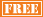January 26
March 9
May 4
June 1

February 9
April 13
June 8

April 20

• ## 2013 GED Test Dates

Varies by State

• ## 2013 AP Exams Test Dates

Calculus AB May 8
Calculus BC May 8
Statistics May 10
Macroeconomics May 16
Microeconomics May 16

# SAT Math

We provide intensive tutoring for students preparing for the mathematics section of the SAT exam. The math section includes two 25 minute parts of 20 questions and 18 questions, and a 20 minute part of 16 questions. There may also be an experimental math part to be completed in 25 minutes. The math questions consist of multiple choice questions and 10 grid in questions.

We utilize a three phase attack to help students properly prepare for the math section of the SAT Exam. During the course of tutoring sessions, we review numerous simulated SAT type problems covering the math topics for which students are responsible. We teach problem solving strategies and techniques which students can apply to specific types of questions on the exam. And we reinforce SAT syllabus math topics which arise during the review of simulated problems for which students are not sufficiently knowledgeable. These 3 components of our SAT prep program provide students with exam problem recognition the day of the exam and the necessary underlying math foundation and strategies to solve problems efficiently and correctly.

We offer a dynamic, intensive program for students preparing for the mathematics section of the SAT Exam. Our concentration and expertise is the math section of the exam. All of our efforts are directed at the one goal of helping students achieve their highest possible score on the math section of the SAT Exam.

• ## One-on-One SAT Math Program includes:

Initial student consultationInitial student assessmentNumber of sessions determined by student

Review of simulated SAT math problems

Review of strategies for attacking SAT math problems

Review of SAT math syllabus

Homework review following each session

• ## Group SAT Math Program includes:

Initial student consultationInitial student assessmentClass size of no more than 20 students

Review of simulated SAT math problems

Review of strategies for attacking SAT math problems

Review of SAT math syllabus